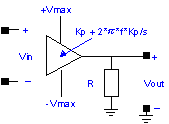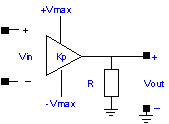﻿ 20-sim webhelp > Library > Iconic Diagrams > Electric > Sources > ControlledVoltageSource

# ControlledVoltageSource

## Library

Iconic Diagrams\Electric\Sources

Voltage

Current

PI

KP

## Use

Domains: Continuous. Size: 1-D. Kind: Iconic Diagrams (Electric).

## Description - Voltage

This model represents an ideal voltage controlled voltage source. Both ports of the model have separate high and low terminals:

p_in.u = p_in_high.u - p_in_low.u;

p_in_high.i = p_in_low.i = p_in.i;

p_out.u = p_out_high.u - p_out_low.u = 0

p_out_high.i = p_out_low.i = p_in.i;

The equations of this model are:

p_in.i = 0;

p_out.u = p_in.u;

p_out.i = indifferent;

## Interface - Voltage

 Ports Description p_in_high, P_in_low p_out_high, P_out_low Both terminals of the input port p_in (Electric). Both terminals of the output port p_out (Electric). Causality fixed current out p_in fixed voltage out p_out

## Description - Current

This model represents an ideal current controlled voltage source. Both ports of the model have separate high and low terminals:

p_in.u = p_in_high.u - p_in_low.u;

p_in_high.i = p_in_low.i = p_in.i;

p_out.u = p_out_high.u - p_out_low.u = 0

p_out_high.i = p_out_low.i = p_in.i;

The equations of this model are:

p_in.u = 0;

p_out.u = p_in.i;

p_out.i = indifferent;

## Interface - Current

 Ports Description p_in_high, P_in_low p_out_high, P_out_low Both terminals of the input port p_in (Electric). Both terminals of the output port p_out (Electric). Causality fixed voltage out p_in fixed voltage out p_out

## Description - PI

This model represents a voltage controlled voltage source. The output voltage is connected to the input voltage through a PI-controller and limited to a maximum and minimum value of ±Vmax. The output voltage is subjected to an output resistance R.Both ports of the model have separate high and low terminals:

p_in.u = p_in_high.u - p_in_low.u;

p_in_high.i = p_in_low.i = p_in.i;

p_out.u = p_out_high.u - p_out_low.u = 0

p_out_high.i = p_out_low.i = p_in.i;

## Interface - PI

 Ports Description p_in_high, p_in_low Both terminals of the input port p_in (Electric). p_out_high, p_out_low Both terminals of the output port p_out (Electric). Causality fixed current out p_in fixed voltage out p_out Parameters Kp f Vmax R Proportional gain [] Integration frequency [Hz] Maximum output voltage [V] Output resistance [Ohm]

## Description - KP

This model represents a voltage controlled voltage source. The output voltage is proportional to the input voltage and is limited to a maximum and minimum value of ±Vmax. The output voltage is subjected to an output resistance R.Both ports of the model have separate high and low terminals:

p_in.u = p_in_high.u - p_in_low.u;

p_in_high.i = p_in_low.i = p_in.i;

p_out.u = p_out_high.u - p_out_low.u = 0

p_out_high.i = p_out_low.i = p_in.i;

## Interface - KP

 Ports Description p_in_high, p_in_low Both terminals of the input port p_in (Electric). p_out_high, p_out_low Both terminals of the output port p_out (Electric). Causality fixed current out p_in fixed voltage out p_out Parameters Kp Vmax R Proportional gain [] Maximum output voltage [V] Output resistance [Ohm]